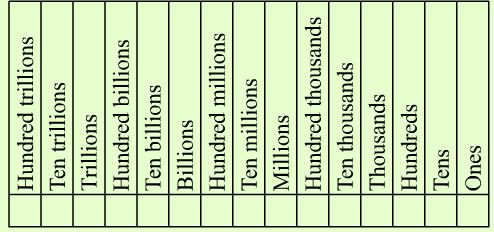### Whole number concepts Math 10

Whole numbers are {0,1,2,3,4,5....}It is very important to know the place values and how to do the 4 operations. (add, subtract, multiply, divide)

This topic also includes:

 word names rounding numbers estimating answers solving applications including finding the average of a set of numbers order of operations express a number with exponents prime or composite numbers prime factorization LCM lowest common multiple

Click on Whole number concepts to try the problems. Have paper and pencil with you to work the answers for the 26 problems.

Whole number concepts math 10